Next: Expressing disjunctive subgoals Up: No Title Previous: Operators

# Unification

The way in which Prolog matches two terms is called unification. The idea is similar to that of unification in logic: we have two terms and we want to see if they can be made to represent the same structure. For example, we might have in our database the single Polog clause:

```parent(alan, clive).
```
and give the query:
```|?- parent(X,Y).
```
We would expect `X` to be instantiated to `alan` and `Y` to be instantiated to `clive` when the query succeeds. We would say that the term `parent(X,Y)` unifies with the term `parent(alan, clive)` with `X` bound to `alan` and `Y` bound to `clive`. The unification algorithm in Prolog is roughly this:

df:un   Given two termsandwhich are to be unified:

• Ifand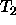are constants (i.e. atoms or numbers) then if they are the same succeed. Otherwise fail.
• Ifis a variable then instantiate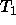to.
• Otherwise, Ifis a variable then instantiateto.
• Otherwise, ifandare complex terms with the same arity (number of arguments), find the principal functor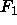ofand principal functor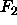of. If these are the same, then take the ordered set of arguments ofofand the ordered set of argumentsof. For each pair of arguments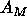and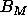from the same position in the term,must unify with.
• Otherwise fail.

For example: applying this procedure to unify `foo(a,X)` with `foo(Y,b)` we get:

• `foo(a,X)` and `foo(Y,b)` are complex terms with the same arity (2).
• The principal functor of both terms is `foo`.
• The arguments (in order) of `foo(a,X)` are `a` and `X`.
• The arguments (in order) of `foo(Y,b)` are `Y` and `b`.
• So `a` and `Y` must unify , and `X` and `b` must unify.
• `Y` is a variable so we instantiate `Y` to `a`.
• `X` is a variable so we instantiate `X` to `b`.
• The resulting term, after unification is `foo(a,b)`.

The built in Prolog operator `'='` can be used to unify two terms. Below are some examples of its use. Annotations are between `**` symbols.

```| ?- a = a.        ** Two identical atoms unify **
yes
| ?- a = b.        ** Atoms don't unify if they aren't identical **
no
| ?- X = a.        ** Unification instantiates a variable to an atom **
X=a
yes
| ?- X = Y.        ** Unification binds two differently named variables **
X=_125451     ** to a single, unique variable name **
Y=_125451
yes
| ?- foo(a,b) = foo(a,b).       ** Two identical complex terms unify **
yes
| ?- foo(a,b) = foo(X,Y).       ** Two complex terms unify if they are **
X=a                        ** of the same arity, have the same principal**
Y=b                        ** functor and their arguments unify **
yes
| ?- foo(a,Y) = foo(X,b).       ** Instantiation of variables may occur **
Y=b                        ** in either of the terms to be unified **
X=a
yes
| ?- foo(a,b) = foo(X,X).       ** In this case there is no unification **
no                              ** because foo(X,X)  must have the same **
** 1st and 2nd arguments **
| ?- 2*3+4 = X+Y.       ** The term 2*3+4 has principal functor + **
X=2*3              ** and therefore unifies with X+Y with X instantiated**
Y=4                ** to 2*3 and Y instantiated to 4 **
yes
| ?- [a,b,c] = [X,Y,Z]. ** Lists unify just like other terms **
X=a
Y=b
Z=c
yes
| ?- [a,b,c] = [X|Y].   ** Unification using the '|' symbol  can be used **
X=a                ** to find the head element, X, and tail list, Y, **
Y=[b,c]            ** of a list **
yes
| ?- [a,b,c] = [X,Y|Z]. ** Unification on lists doesn't have to be **
X=a                ** restricted to finding the first head element **
Y=b                ** In this case we find the 1st and 2nd elements **
Z=[c]              ** (X and Y) and then the tail list (Z) **
yes
| ?- [a,b,c] = [X,Y,Z|T].       ** This is a similar example but here **
X=a                        ** the first 3 elements are unified with **
Y=b                        ** variables X, Y and Z, leaving the **
Z=c                        ** tail, T, as an empty list [] **
T=[]
yes
| ?- [a,b,c] = [a|[b|[c|[]]]].  ** Prolog is quite happy to unify these **
yes                             ** because they are just notational **
** variants of the same Prolog term **
```Next: Expressing disjunctive subgoals Up: No Title Previous: Operators

Dave Stuart Robertson
Tue Jul 7 10:44:26 BST 1998